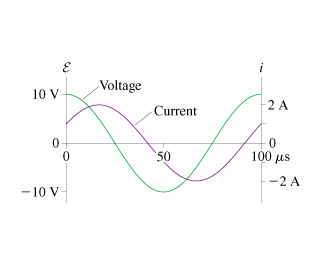# Problem: The figure shows voltage and current graphs for a series RLC circuit.If L = 380μH , what is the resonance frequency?

###### FREE Expert Solution

Phase constant:

Resonance Frequency,

$\overline{){{\mathbf{\omega }}}_{\mathbf{r}\mathbf{e}\mathbf{s}}{\mathbf{=}}\frac{\mathbf{1}}{\sqrt{\mathbf{L}\mathbf{C}}}}$

Impedance,

$\overline{){{\mathbf{X}}}_{{\mathbf{L}}}{\mathbf{=}}{\mathbf{\omega }}{\mathbf{L}}}$

$\overline{){{\mathbf{X}}}_{{\mathbf{C}}}{\mathbf{=}}\frac{\mathbf{1}}{\mathbf{\omega C}}}$

Angular frequency,

$\overline{){\mathbf{\omega }}{\mathbf{=}}{\mathbf{2}}{\mathbf{\pi }}{\mathbf{f}}}$

(2)

Φ = 60°

R = 2.5 Ω

Rearranging the phase constant equation:

R tan Φ = (XL - XC)

99% (83 ratings)###### Problem Details

The figure shows voltage and current graphs for a series RLC circuit.If L = 380μH , what is the resonance frequency?